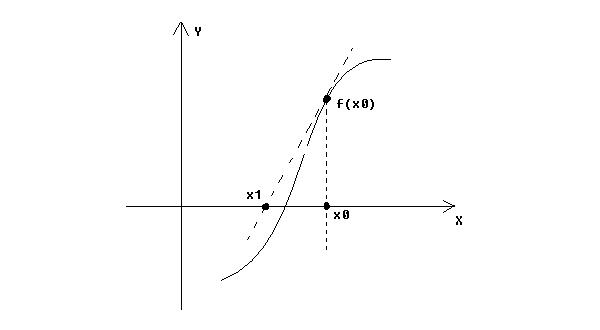Online JudgeProblem SetAuthorsOnline ContestsUser
Web Board
F.A.Qs
Statistical Charts
Problems
Submit Problem
Online Status
Prob.ID:
Register
Authors ranklist
Current Contest
Past Contests
Scheduled Contests
Award Contest
Register
Language:
Newton’s Method
 Time Limit: 1000MS Memory Limit: 65536K Total Submissions: 1114 Accepted: 171

Description

While solving non-linear equations in numerical analysis lesson, professor Busoniya introduce us Newton's Method. Like the other ways (such as bisection, Muller's Method), this method is also based on a linear approximation of the function, but does so using a tangent to the curve. The figure below gives a graphical description.Starting from a single initial estimate, x0, which is not too far from a root, we move along the tangent to its intersection with the x-axis, and take that as the next approximation x1. The general term is: xn+1 = xn - f(xn) / f′(xn), n = 0, 1, 2, .... Newton’s algorithm is widely used because it is more rapidly convergent than any of the methods discussed so far. Then now, you task is to accurate how many iterations it takes to get a root using Newton's Method. The x0 and the equation are given and all the tolerance is 0.000001. You just use this method to get the answer. The iteration will stop when |xn+1 - xn| < tolerance or |f(xn)| < tolerance then n is the answer. But sometimes the x0 or equation we choose is so bad that we cannot get the answer using this method within 1000 iterations, then just output "Bad x0 or bad equation!"

Input

There are multiple cases ended by EOF. Each case has two lines, first line is the equation and the second line is x0. We ensure that each equation just contain integers, 'x', '+', '-', '=' and '^', the right part of '=' is always 0, and there is a space between each term. The number of terms in the equation is less than 50 and all integers in the equations are less than 1000.

Output

For each test case, output n or "Bad x0 or bad equation!" per line.

Sample Input

```-3x^2 -3 = 0
3
3x -3 = 0
1
5x^2 -2 = 0
-5
```

Sample Output

```Bad x0 or bad equation!
0
6
```

Source

[Submit]   [Go Back]   [Status]   [Discuss]Home PageGo BackTo top